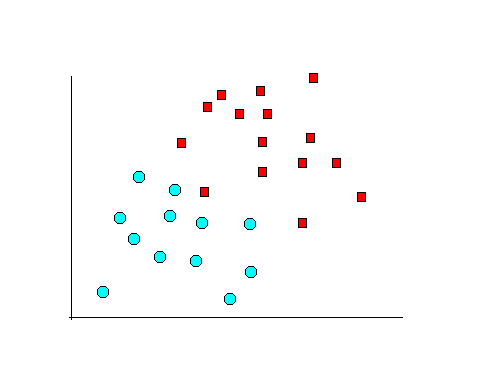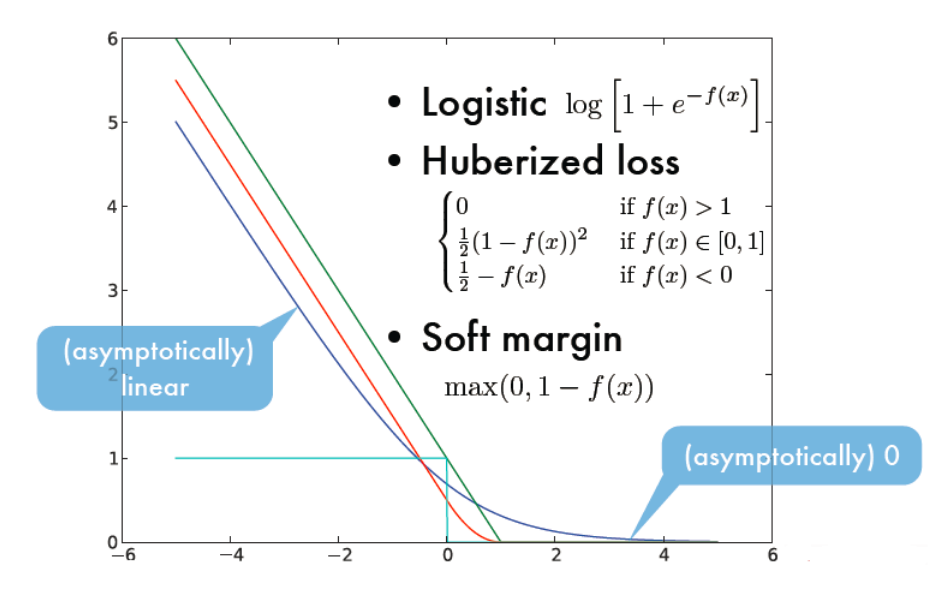Soft Marginyi(θTxi+b)1

minθ,b12||θ||2+Ci=1p0/1(yi(θTxi+b)1)

• hinge损失:hinge(z)=max(0,1z)$\ell_{hinge}(z)=max(0,1-z)$
• 指数损失(exponential loss):exp(z)=exp(z)$\ell_{exp}(z)=exp(-z)$
• 对率损失(logistic loss):log(1+expz)$\ell_{log}(1+exp{-z})$minθ,b12||θ||2+Ci=1pmax(0,1(yi(θTxi+b))

minθ,b,ξi12||θ||2+C1pξis.t.　yi(θTxi+b)1ξiξi0,　i=1,2,...,p

Regularization

minf　Ω(f)+Ci=1p(f(xi),yi)

Ω(f)$\Omega(f)$叫做“结构风险”（structural risk），用与描述模型f的某些性质，一般成为正则化项，表述我们希望获得具有何种性质的模型（例如希望获得复杂度较小的模型），这为引入领域知识和用户意图提供了途径。C称为正则化常数，平衡结构风险和经验风险。Lp$L_p$ 范数是常用的正则化项，其中L2$L_2$范数倾向与θ$\theta$的分量取值尽量均衡，即非零分量个数尽量稠密，而L0$L_0$L1$L_1$范数则倾向于θ$\theta$的分量尽量稀疏，即非零个数尽量少。